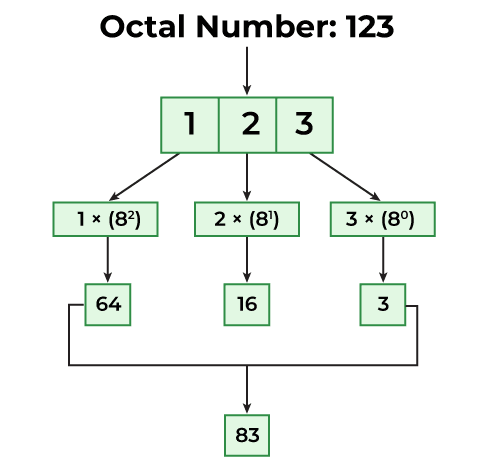Open In App
Related Articles
• Write an Interview Experience
• Java Programs – Java Programming Examples

# Java Program to Convert Octal to Decimal

The octal numbers are numbers with 8 bases and use digits from 0-7. This system is a base 8 number system. The decimal numbers are the numbers with 10 as their base and use digits from 0-9 to represent the decimal number. They also require dots to represent decimal fractions.

We have to convert a number that is in the Octal Number System to the Decimal Number System. The base in an Octal Number is 8, which means that an Octal Number will have digits ranging from 0 to 7.

#### For Example:

In Octal: 167

In Decimal:(7 * 80) + (6 * 81) +(1 * 82)=119

The below diagram explains how to convert an octal number (123) to an equivalent decimal value:## 1. Using Integer.parseInt() method

To convert any string form to decimal, we can use type.parseType() method. For example, here we need to convert from octal to decimal, and the octal form is an integer, so we can use Integer.parseInt() to convert it.

## Java

 `// Java program to convert``// octal number to decimal using``// Integer.parseInt()` `public` `class` `GFG {` `    ``public` `static` `void` `main(String args[])``    ``{``        ``// octal value``        ``String onum = ``"157"``;` `        ``// octal to decimal using Integer.parseInt()``        ``int` `num = Integer.parseInt(onum, ``8``);` `        ``System.out.println(``            ``"Decimal equivalent of Octal value 157 is: "``            ``+ num);``    ``}``}`

Output

```Decimal equivalent of Octal value 157 is: 111
```

#### The complexity of the above method:

Time complexity : O(1)
Auxiliary space : O(1)

## 2. Custom Method to Convert Octal to Decimal

### Algorithm

1. Start and take the octal input from the user.
2. Create a result variable with an initial value of 0 to store the resultant Decimal number.
3. Create a loop for getting every digit in the Input.
4. Multiply each digit in the number with 8n-1, where n is the digit’s position.
5. Then add it to the result.
6. Store the value in Step(5) to the result variable.
7. Print the result variable.

## Java

 `// Java program to convert octal``// to decimal number using custom code``import` `java.lang.Math;` `// Driver Class``public` `class` `Main {``    ``// main function``    ``public` `static` `void` `main(String[] args)``    ``{``        ``int` `a = ``167``;` `        ``// Initialize result variable to 0.``        ``int` `result = ``0``;` `        ``// Take a copy of input``        ``int` `copy_a = a;` `        ``for` `(``int` `i = ``0``; copy_a > ``0``; i++) {` `            ``// Take the last digit``            ``int` `temp = copy_a % ``10``;` `            ``// Appropriate power on 8 suitable to``            ``// its position.``            ``double` `p = Math.pow(``8``, i);` `            ``// Multiply the digits to the  into the Input``            ``// and``            ``//  then add it to result``            ``result += (temp * p);``            ``copy_a = copy_a / ``10``;``        ``}` `        ``System.out.print(``"Decimal of Octal Number ("` `+ a``                         ``+ ``") : "` `+ result);``    ``}``}`

Output

`Decimal of Octal Number (167) : 119`

#### The complexity of the above method:

Time complexity: O(logN) for given octal number N